# Cartesian coordinate system

Cartesian coordinate system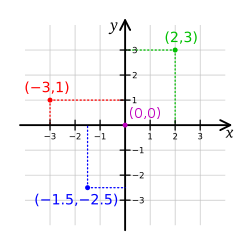Illustration of a Cartesian coordinate plane. Four points are marked and labeled with their coordinates: (2, 3) in green, (−3, 1) in red, (−1.5, −2.5) in blue, and the origin (0, 0) in purple.

A Cartesian coordinate system specifies each point uniquely in a plane by a pair of numerical coordinates, which are the signed distances from the point to two fixed perpendicular directed lines, measured in the same unit of length. Each reference line is called a coordinate axis or just axis of the system, and the point where they meet is its origin, usually at ordered pair (0,0). The coordinates can also be defined as the positions of the perpendicular projections of the point onto the two axes, expressed as signed distances from the origin.

One can use the same principle to specify the position of any point in three-dimensional space by three Cartesian coordinates, its signed distances to three mutually perpendicular planes (or, equivalently, by its perpendicular projection onto three mutually perpendicular lines). In general, one can specify a point in a space of any dimension n by use of n Cartesian coordinates, the signed distances from n mutually perpendicular hyperplanes.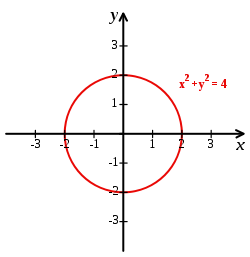Cartesian coordinate system with a circle of radius 2 centered at the origin marked in red. The equation of a circle is (x - a)2 + (y - b)2 = r2 where a and b are the coordinates of the center (a, b) and r is the radius.

The invention of Cartesian coordinates in the 17th century by René Descartes (Latinized name: Cartesius) revolutionized mathematics by providing the first systematic link between Euclidean geometry and algebra. Using the Cartesian coordinate system, geometric shapes (such as curves) can be described by Cartesian equations: algebraic equations involving the coordinates of the points lying on the shape. For example, a circle of radius 2 may be described as the set of all points whose coordinates x and y satisfy the equation x2 + y2 = 4.

Cartesian coordinates are the foundation of analytic geometry, and provide enlightening geometric interpretations for many other branches of mathematics, such as linear algebra, complex analysis, differential geometry, multivariate calculus, group theory, and more. A familiar example is the concept of the graph of a function. Cartesian coordinates are also essential tools for most applied disciplines that deal with geometry, including astronomy, physics, engineering, and many more. They are the most common coordinate system used in computer graphics, computer-aided geometric design, and other geometry-related data processing.

## History

The adjective Cartesian refers to the French mathematician and philosopher René Descartes (who used the name Cartesius in Latin).

The idea of this system was developed in 1637 in two writings by Descartes and independently by Pierre de Fermat, although Fermat used three dimensions, and did not publish the discovery. Descartes introduces the new idea of specifying the position of a point or object on a surface, using two intersecting axes as measuring guides.[citation needed] In La Géométrie, he further explores the above-mentioned concepts.

The development of the Cartesian coordinate system enabled the development of perspective and projective geometry. It would later play an intrinsic role in the development of calculus by Isaac Newton and Gottfried Wilhelm Leibniz.

Nicole Oresme, a French philosopher of the 14th Century, used constructions similar to Cartesian coordinates well before the time of Descartes.

Many other coordinate systems have been developed since Descartes, such as the polar coordinates for the plane, and the spherical and cylindrical coordinates for three-dimensional space.

## Definitions

### Number line

Choosing a Cartesian coordinate system for a one-dimensional space—that is, for a straight line—means choosing a point O of the line (the origin), a unit of length, and an orientation for the line. An orientation chooses which of the two half-lines determined by O is the positive, and which is negative; we then say that the line "is oriented" (or "points") from the negative half towards the positive half, where the direction is that of counting. Then each point p of the line can be specified by its distance from O, taken with a + or − sign depending on which half-line contains p.

A line with a chosen Cartesian system is called a number line. Every real number, whether integer, rational, or irrational, has a unique location on the line. Conversely, every point on the line can be interpreted as a number in an ordered continuum which includes the real numbers.

### Cartesian coordinates in two dimensions

The modern Cartesian coordinate system in two dimensions (also called a rectangular coordinate system) is defined by an ordered pair of perpendicular lines (axes), a single unit of length for both axes, and an orientation for each axis. (Early systems allowed "oblique" axes, that is, axes that did not meet at right angles.) The lines are commonly referred to as the x and y-axes where the x-axis is taken to be horizontal and the y-axis is taken to be vertical. The point where the axes meet is taken as the origin for both, thus turning each axis into a number line. For a given point P, a line is drawn through P perpendicular to the x-axis to meet it at X and second line is drawn through P perpendicular to the y-axis to meet it at Y. The coordinates of P are then X and Y interpreted as numbers x and y on the corresponding number lines. The coordinates are written as an ordered pair (xy).

The point where the axes meet is the common origin of the two number lines and is simply called the origin. It is often labeled O and if so then the axes are called Ox and Oy. A plane with x and y-axes defined is often referred to as the Cartesian plane or xy plane. The value of x is called the x-coordinate or abscissa and the value of y is called the y-coordinate or ordinate.

The choices of letters come from the original convention, which is to use the latter part of the alphabet to indicate unknown values. The first part of the alphabet was used to designate known values.

### Cartesian coordinates in three dimensions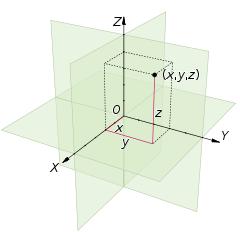A three dimensional Cartesian coordinate system, with origin O and axis lines X, Y and Z, oriented as shown by the arrows. The tick marks on the axes are one length unit apart. The black dot shows the point with coordinates X = 2, Y = 3, and Z = 4, or (2,3,4).

Choosing a Cartesian coordinate system for a three-dimensional space means choosing an ordered triplet of lines (axes), any two of them being perpendicular; a single unit of length for all three axes; and an orientation for each axis. As in the two-dimensional case, each axis becomes a number line. The coordinates of a point p are obtained by drawing a line through p perpendicular to each coordinate axis, and reading the points where these lines meet the axes as three numbers of these number lines.

Alternatively, the coordinates of a point p can also be taken as the (signed) distances from p to the three planes defined by the three axes. If the axes are named x, y, and z, then the x coordinate is the distance from the plane defined by the y and z axes. The distance is to be taken with the + or − sign, depending on which of the two half-spaces separated by that plane contains p. The y and z coordinates can be obtained in the same way from the (x,z) and (x,y) planes, respectively.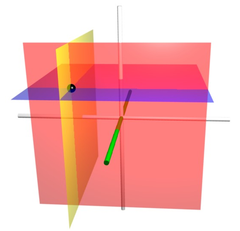The coordinate surfaces of the Cartesian coordinates (x, y, z). The z-axis is vertical and the x-axis is highlighted in green. Thus, the red plane shows the points with x=1, the blue plane shows the points with z=1, and the yellow plane shows the points with y=-1. The three surfaces intersect at the point P (shown as a black sphere) with the Cartesian coordinates (1, -1, 1).

### Generalizations

One can generalize the concept of Cartesian coordinates to allow axes that are not perpendicular to each other, and/or different units along each axis. In that case, each coordinate is obtained by projecting the point onto one axis along a direction that is parallel to the other axis (or, in general, to the hyperplane defined by all the other axes). In those oblique coordinate systems the computations of distances and angles is more complicated than in standard Cartesian systems, and many standard formulas (such as the Pythagorean formula for the distance) do not hold.

## Notations and conventions

The Cartesian coordinates of a point are usually written in parentheses and separated by commas, as in (10,5) or (3,5,7). The origin is often labelled with the capital letter O. In analytic geometry, unknown or generic coordinates are often denoted by the letters x and y on the plane, and x, y, and z in three-dimensional space. w is often used for four-dimensional space, but the rarity of such usage precludes concrete convention here. This custom comes from an old convention of algebra, to use letters near the end of the alphabet for unknown values (such as were the coordinates of points in many geometric problems), and letters near the beginning for given quantities.

These conventional names are often used in other domains, such as physics and engineering. However, other letters may be used too. For example, in a graph showing how a pressure varies with time, the graph coordinates may be denoted t and P. Each axis is usually named after the coordinate which is measured along it; so one says the x-axis, the y-axis, the t-axis, etc.

Another common convention for coordinate naming is to use subscripts, as in x1, x2, ... xn for the n coordinates in an n-dimensional space; especially when n is greater than 3, or variable. Some authors (and many programmers) prefer the numbering x0, x1, ... xn−1. These notations are especially advantageous in computer programming: by storing the coordinates of a point as an array, instead of a record, one can use iterative commands or procedure parameters instead of repeating the same commands for each coordinate.

In mathematical illustrations of two-dimensional Cartesian systems, the first coordinate (traditionally called the abscissa) is measured along a horizontal axis, oriented from left to right. The second coordinate (the ordinate) is then measured along a vertical axis, usually oriented from bottom to top.

However, in computer graphics and image processing one often uses a coordinate system with the y axis pointing down (as displayed on the computer's screen). This convention developed in the 1960s (or earlier) from the way that images were originally stored in display buffers.

For three-dimensional systems, the z axis is often shown vertical and pointing up (positive up), so that the x and y axes lie on a horizontal plane. If a diagram (3D projection or 2D perspective drawing) shows the x and y axis horizontally and vertically, respectively, then the z axis should be shown pointing "out of the page" towards the viewer or camera. In such a 2D diagram of a 3D coordinate system, the z axis would appear as a line or ray pointing down and to the left or down and to the right, depending on the presumed viewer or camera perspective. In any diagram or display, the orientation of the three axes, as a whole, is arbitrary. However, the orientation of the axes relative to each other should always comply with the right-hand rule, unless specifically stated otherwise. All laws of physics and math assume this right-handedness, which ensures consistency. For 3D diagrams, the names "abscissa" and "ordinate" are rarely used for x and y, respectively. When they are, the z-coordinate is sometimes called the applicate.

The words abscissa, ordinate and applicate are sometimes used to refer to coordinate axes rather than values.The four quadrants of a Cartesian coordinate system.

The axes of a two-dimensional Cartesian system divide the plane into four infinite regions, called quadrants, each bounded by two half-axes. These are often numbered from 1st to 4th and denoted by Roman numerals: I (where the signs of the two coordinates are I (+,+), II (−,+), III (−,−), and IV (+,−). When the axes are drawn according to the mathematical custom, the numbering goes counter-clockwise starting from the upper right ("northeast") quadrant.

Similarly, a three-dimensional Cartesian system defines a division of space into eight regions or octants, according to the signs of the coordinates of the points. The octant where all three coordinates are positive is sometimes called the first octant; however, there is no established nomenclature for the other octants. The n-dimensional generalization of the quadrant and octant is the orthant.

## Cartesian space

A Euclidean plane with a chosen Cartesian system is called a Cartesian plane. Since Cartesian coordinates are unique and non-ambiguous, the points of a Cartesian plane can be identified with all possible pairs of real numbers; that is with the Cartesian product$\R^2 = \R\times\R$, where$\R$ is the set of all reals. In the same way one defines a Cartesian space of any dimension n, whose points can be identified with the tuples (lists) of n real numbers, that is, with$\R^n$.

## Cartesian formulas for the plane

### Distance between two points

The Euclidean distance between two points of the plane with Cartesian coordinates (x1,y1) and (x2,y2) is$d = \sqrt{(x_2-x_1)^2 + (y_2-y_1)^2}.$

This is the Cartesian version of Pythagoras' theorem. In three-dimensional space, the distance between points (x1,y1,z1) and (x2,y2,z2) is$d = \sqrt{(x_2-x_1)^2 + (y_2-y_1)^2+ (z_2-z_1)^2}$

which can be obtained by two consecutive applications of Pythagoras' theorem.

### Euclidean transformations

#### Translation

Translating a set of points of the plane, preserving the distances and directions between them, is equivalent to adding a fixed pair of numbers (X,Y) to the Cartesian coordinates of every point in the set. That is, if the original coordinates of a point are (x,y), after the translation they will be$(x',y') = (x + X, y + Y).\,$

#### Scaling

To make a figure larger or smaller is equivalent to multiplying the Cartesian coordinates of every point by the same positive number m. If (x,y) are the coordinates of a point on the original figure, the corresponding point on the scaled figure has coordinates$(x',y') = (m x, m y).\,$

If m is greater than 1, the figure becomes larger; if m is between 0 and 1, it becomes smaller.

#### Rotation

To rotate a figure counterclockwise around the origin by some angle θ is equivalent to replacing every point with coordinates (x,y) by the point with coordinates (x',y'), where$x'=x \cos \theta - y \sin \theta\,$$y'=x \sin \theta + y \cos \theta.\,$

Thus:$(x',y') = ((x \cos \theta - y \sin \theta\,) , (x \sin \theta + y \cos \theta\,)).$

#### Reflection

If (x, y) are the Cartesian coordinates of a point, then (−x, y) are the coordinates of its reflection across the second coordinate axis (the Y axis), as if that line were a mirror. Likewise, (x, −y) are the coordinates of its reflection across the first coordinate axis (the X axis).

#### General transformations

The Euclidean transformations of the plane are the translations, rotations, scalings, reflections, and arbitrary compositions thereof. The result (x',y') of applying a Euclidean transformation to a point (x,y) is given by the formula$(x',y') = (x,y) A + b\,$

where A is a 2×2 matrix and b is a pair of numbers, that depend on the transformation; that is,$x' = x A_{1 1} + y A_{2 1} + b_{1}\,$$y' = x A_{1 2} + y A_{2 2} + b_{2}.\,$

The matrix A must have orthogonal rows with same Euclidean length, that is,$A_{1 1} A_{2 1} + A_{1 2} A_{2 2} = 0\,$

and$A_{1 1}^2 + A_{1 2}^2 = A_{2 1}^2 + A_{2 2}^2.$

This is equivalent to saying that A times its transpose must be a diagonal matrix. If these conditions do not hold, the formula describes a more general affine transformation of the plane.

The formulas define a translation if and only if A is the identity matrix. The transformation is a rotation around some point if and only if A is a rotation matrix, meaning that$A_{1 1}^2 + A_{1 2}^2 = A_{2 1}^2 + A_{2 2}^2 = A_{1 1} A_{2 2} - A_{2 1} A_{1 2} = 1.$

## Orientation and handedness

### In two dimensions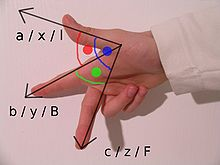The right hand rule.

Fixing or choosing the x-axis determines the y-axis up to direction. Namely, the y-axis is necessarily the perpendicular to the x-axis through the point marked 0 on the x-axis. But there is a choice of which of the two half lines on the perpendicular to designate as positive and which as negative. Each of these two choices determines a different orientation (also called handedness) of the Cartesian plane.

The usual way of orienting the axes, with the positive x-axis pointing right and the positive y-axis pointing up (and the x-axis being the "first" and the y-axis the "second" axis) is considered the positive or standard orientation, also called the right-handed orientation.

A commonly used mnemonic for defining the positive orientation is the right hand rule. Placing a somewhat closed right hand on the plane with the thumb pointing up, the fingers point from the x-axis to the y-axis, in a positively oriented coordinate system.

The other way of orienting the axes is following the left hand rule, placing the left hand on the plane with the thumb pointing up.

When pointing the thumb away from the origin along an axis, the curvature of the fingers indicates a positive rotation along that axis.

Regardless of the rule used to orient the axes, rotating the coordinate system will preserve the orientation. Switching any two axes will reverse the orientation.

### In three dimensionsFig. 7 – The left-handed orientation is shown on the left, and the right-handed on the right.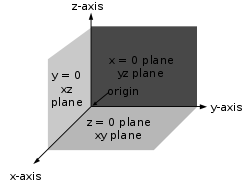Fig. 8 – The right-handed Cartesian coordinate system indicating the coordinate planes.

Once the x- and y-axes are specified, they determine the line along which the z-axis should lie, but there are two possible directions on this line. The two possible coordinate systems which result are called 'right-handed' and 'left-handed'. The standard orientation, where the xy-plane is horizontal and the z-axis points up (and the x- and the y-axis form a positively oriented two-dimensional coordinate system in the xy-plane if observed from above the xy-plane) is called right-handed or positive.

The name derives from the right-hand rule. If the index finger of the right hand is pointed forward, the middle finger bent inward at a right angle to it, and the thumb placed at a right angle to both, the three fingers indicate the relative directions of the x-, y-, and z-axes in a right-handed system. The thumb indicates the x-axis, the index finger the y-axis and the middle finger the z-axis. Conversely, if the same is done with the left hand, a left-handed system results.

Figure 7 depicts a left and a right-handed coordinate system. Because a three-dimensional object is represented on the two-dimensional screen, distortion and ambiguity result. The axis pointing downward (and to the right) is also meant to point towards the observer, whereas the "middle" axis is meant to point away from the observer. The red circle is parallel to the horizontal xy-plane and indicates rotation from the x-axis to the y-axis (in both cases). Hence the red arrow passes in front of the z-axis.

Figure 8 is another attempt at depicting a right-handed coordinate system. Again, there is an ambiguity caused by projecting the three-dimensional coordinate system into the plane. Many observers see Figure 8 as "flipping in and out" between a convex cube and a concave "corner". This corresponds to the two possible orientations of the coordinate system. Seeing the figure as convex gives a left-handed coordinate system. Thus the "correct" way to view Figure 8 is to imagine the x-axis as pointing towards the observer and thus seeing a concave corner.

## Representing a vector in the standard basis

A point in space in a Cartesian coordinate system may also be represented by a vector, which can be thought of as an arrow pointing from the origin of the coordinate system to the point. If the coordinates represent spatial positions (displacements) it is common to represent the vector from the origin to the point of interest as$\mathbf{r}$. In three dimensions, the vector from the origin to the point with Cartesian coordinates (x,y,z) is sometimes written as:$\mathbf{r} = x \mathbf{i} + y \mathbf{j} + z \mathbf{k}$

where$\mathbf{i}$,$\mathbf{j}$, and$\mathbf{k}$ are unit vectors and the respective versors of x, y, and z axes. This is the quaternion representation of the vector, and was introduced by Sir William Rowan Hamilton. The unit vectors$\mathbf{i}$,$\mathbf{j}$, and$\mathbf{k}$ are called the versors of the coordinate system, and are the vectors of the standard basis in three-dimensions.

## Applications

Each axis may have different units of measurement associated with it (such as kilograms, seconds, pounds, etc.). Although four- and higher-dimensional spaces are difficult to visualize, the algebra of Cartesian coordinates can be extended relatively easily to four or more variables, so that certain calculations involving many variables can be done. (This sort of algebraic extension is what is used to define the geometry of higher-dimensional spaces.) Conversely, it is often helpful to use the geometry of Cartesian coordinates in two or three dimensions to visualize algebraic relationships between two or three of many non-spatial variables.

The graph of a function or relation is the set of all points satisfying that function or relation. For a function of one variable, f, the set of all points (x,y) where y = f(x) is the graph of the function f. For a function of two variables, g, the set of all points (x,y,z) where z = g(x,y) is the graph of the function g. A sketch of the graph of such a function or relation would consist of all the salient parts of the function or relation which would include its relative extrema, its concavity and points of inflection, any points of discontinuity and its end behavior. All of these terms are more fully defined in calculus. Such graphs are useful in calculus to understand the nature and behavior of a function or relation.

Wikimedia Foundation. 2010.

Поможем решить контрольную работу

### Look at other dictionaries:

• Cartesian coordinate system — noun a coordinate system for which the coordinates of a point are its distances from a set perpendicular lines that intersect at the origin of the system • Hypernyms: ↑coordinate system, ↑frame of reference, ↑reference system, ↑reference frame …   Useful english dictionary

• Coordinate system — For geographical coordinates on Wikipedia, see Wikipedia:WikiProject Geographical coordinates. In geometry, a coordinate system is a system which uses one or more numbers, or coordinates, to uniquely determine the position of a point or other… …   Wikipedia

• coordinate system — noun a system that uses coordinates to establish position • Syn: ↑frame of reference, ↑reference system, ↑reference frame • Hypernyms: ↑arrangement, ↑organization, ↑organisation, ↑system …   Useful english dictionary

• Polar coordinate system — Points in the polar coordinate system with pole O and polar axis L. In green, the point with radial coordinate 3 and angular coordinate 60 …   Wikipedia

• Cylindrical coordinate system — A cylindrical coordinate system with origin O, polar axis A, and longitudinal axis L. The dot is the point with radial distance ρ = 4, angular coordinate φ = 130°, and height z = 4. A cylindrical coordinate system is …   Wikipedia

• Spherical coordinate system — In mathematics, the spherical coordinate system is a coordinate system for representing geometric figures in three dimensions using three coordinates: the radial distance of a point from a fixed origin, the zenith angle from the positive z axis… …   Wikipedia

• Cartesian coordinate — noun one of the coordinates in a system of coordinates that locates a point on a plane or in space by its distance from two lines or three planes respectively; the two lines or the intersections of the three planes are the coordinate axes •… …   Useful english dictionary

• coordinate system — Math. any method that uses numbers to represent a point, line, or the like. * * * Arrangement of reference lines or curves used to identify the location of points in space. In two dimensions, the most common system is the Cartesian (after René… …   Universalium

• cartesian coordinate — /kaˌtiʒən koʊˈɔdənət/ (say kah.teezhuhn koh awduhnuht) noun Mathematics one of the set of numbers that locate a point in a rectilinear two dimensional (or three dimensional) system, defined by the perpendicular distances of the point from two (or …   Australian-English dictionary

• State Plane Coordinate System — The State Plane Coordinate System (SPS or SPCS) is a set of 126 geographic zones or coordinate systems designed for specific regions of the United States. Each state contains one or more state plane zones, the boundaries of which usually follow… …   Wikipedia